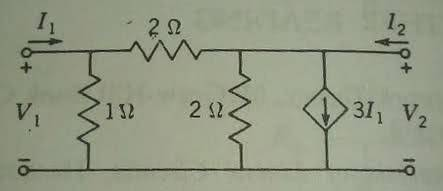# 2 port network Y parameters

for the following circuit, i am trying to find the y parametersi have found y11, y12, and y22 to match the answer that my text provides, but i am having trouble with y21

the solution given is y11 = 1.5, y12 = -0.5, y21 = 4, y22 = -0.5

in trying to solve for y21, i get 4.5
this is what i attempted:
short the voltage source at port 2
I1 = (v1 - va)/2 - v1
= -0.5(v1/2 - 3I1 - v1) - v1
= -0.5(-0.5v1 - 3I1) - v1
= 0.25v1 + 1.5I1 - v1
-0.5I1 = -0.75v1
I2 = 3I1
I1 = (1/3)I2
-0.5((1/3)I2) = -0.75v1
y21 = I2/v1 = 18/4 = 4.5

rude man
Homework Helper
Gold Member
for the following circuit, i am trying to find the y parametersi have found y11, y12, and y22 to match the answer that my text provides, but i am having trouble with y21

the solution given is y11 = 1.5, y12 = -0.5, y21 = 4, y22 = -0.5

in trying to solve for y21, i get 4.5
this is what i attempted:
short the voltage source at port 2
I1 = (v1 - va)/2 - v1
= -0.5(v1/2 - 3I1 - v1) - v1
= -0.5(-0.5v1 - 3I1) - v1
= 0.25v1 + 1.5I1 - v1
-0.5I1 = -0.75v1
I2 = 3I1
I1 = (1/3)I2
-0.5((1/3)I2) = -0.75v1
y21 = I2/v1 = 18/4 = 4.5

1. What is Va? Looks like you meant Va = output = 0, in which case your 1st equation is incorrect.
2. I suggest writing an expression for the sum of currents at the output = 0 to start.

Va is the node voltage between the two 2-Ohm resistors. why is the first equation incorrect?

rude man
Homework Helper
Gold Member
Va is the node voltage between the two 2-Ohm resistors. why is the first equation incorrect?

What happened to your schematic? It's been removed!

So I don't know for sure which node you're talking about. But since there are,as I recall, only two- is Va the output node? If it is, why call it Va when it's in fact V2?

Last edited:i obtained the correct answer by transforming the dependent current source into a voltage source in series with the 2 ohm resistor. i was doing something wrong in the node method. i'm still not sure what it is.

rude man
Homework Helper
Gold Member
That's a perfectly good way to do it.

Labeling the resistors R1, R2, R3 from left to right and summing currents at the output:

V1/R2 + I2 = 3I1
V1/R2 + I2 = 3V1/R where R = R1||R2
V1/2 + I2 = 3V1/(2/3) = 9V1/2
I2 = 9V1/2 - V1/2 = 8V1/2 = 4V1
I2/V1 = 4

That's a perfectly good way to do it.

Labeling the resistors R1, R2, R3 from left to right and summing currents at the output:

V1/R2 + I2 = 3I1
V1/R2 + I2 = 3V1/R where R = R1||R2
V1/2 + I2 = 3V1/(2/3) = 9V1/2
I2 = 9V1/2 - V1/2 = 8V1/2 = 4V1
I2/V1 = 4

thanks for that. i just realized now you had said Va = 0 according to the way i had defined it. that cleared things up.

The Electrician
Gold Member
i was doing something wrong in the node method. i'm still not sure what it is.

Your assumption that I2 = 3I1 was wrong.

The Y parameters for a two port like you show are defined like this:

y11 V1 + y12 V2 = I1
y21 V1 + y22 V2 = I2

If we delete the dependent source, it's easy to derive the Y parameters for the resistors only:

1.5 V1 - .5 V2 = I1
-.5 V1 + 1 V2 = I2

In other words:

y11 = 1.5
y12 = -.5
y21 = -.5
y22 = 1

If we go back to the general Y parameter equations:

y11 V1 + y12 V2 = I1
y21 V1 + y22 V2 = I2

We can simply add the dependent source to the right side of the second equation since it's a current source applied to the V2 node:

y11 V1 + y12 V2 = I1
y21 V1 + y22 V2 = I2-3*I1

We see that the first equation tells us that I1 = y11 V1 + y12 V2, so our equations become:

y11 V1 + y12 V2 = I1
y21 V1 + y22 V2 = I2-3*(y11 V1 + y12 V2)

which becomes:

y11 V1 + y12 V2 = I1
(3 y11+y21) V1 + (3 y12+y22) V2 = I2

or we could write them as:

y11 V1 + y12 V2 = I1
y'21 V1 + y'22 V2 = I2

with y'21 representing the y21 parameter after the dependent source is added.

Substituting numerical values:

1.5 V1 - .5 V2 = I1
(3*1.5-.5) V1 + (3*(-.5)+1) V2 = I2

and finally:

1.5 V1 - .5 V2 = I1
4 V1 -.5 V2 = I2